| my account | login-logout | resources | classroom help | support | catalog | home | get webcard |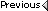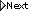» Online Classroom   »   » Public Discussion of Cel Nav   » Clarification of algorithms for use with Cel Nav

Author Topic: Clarification of algorithms for use with Cel Nav
 Al Szymanski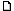posted October 28, 2009 01:00 PM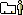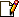Good day. ( Thanks David for letting me back in ).A number of years ago, I took the at home Celestial Navigation course and was quite pleased with the product and my advancement.I went on to take a hand at doing lunars and getting into the core math and theory on my own.As I said, this was a number of years ago.I have a new problem, that I am confident that you folks can drop a bit of help my way.I am trying to do the Law of Cosines method for calculation Hc and Z for a given set of locations.I have the calculation of Hc down pretty confidently using the following algorithm:Hc = arccos ( cos ( 90 - Lat ) * cos ( 90 - Dec ) + sin ( 90 - Lat ) * sin ( 90 - Dec ) * cos ( LHA ) )- and -Hc = arccos ( sin ( Lat ) * sin ( Dec ) + cos ( Lat ) * cos ( Dec ) * cos ( LHA ) )However it is my attempts at getting Zc that are failing.I have two equations; both fail on my calculator by trying to perform an arccos of a number greater than 1.Zc = arccos ( ( sin ( 90 - Dec ) - ( sin ( 90 - Lat ) * sin ( Hc ) ) / ( cos ( 90 - Lat ) * cos ( Hc ) ) )Zc = arccos ( ( sin ( Dec ) - ( sin ( Lat ) * cos ( Hc ) ) ) / ( cos ( Lat ) * cos ( Hc ) )I know that they cannot be both correct, but both come from reputable resources and both are failing.Last question : both examples of these formulae do not state whether or not the angles need be in radians or if they may be in degrees. I am wondering if this is the entire crux of the problem. Thanks again. Al
 David Burchposted October 28, 2009 07:23 PMPlease check our online glossary for cel nav at www.starpath.com/glossary, and look under the term Navigator's triangle and you will find the right equation. It seems that your two options are each slightly off of what we have, which i believe is correct.a shortcut to the answerPS, normally it does not matter what the angles are. I would use degrees only. You only have to use radians if you using say Visual Basic programming or doing it in a spread sheet. hand held calculators let you choose degrees or radians. From: Starpath, Seattle, WA
 HHEWposted October 28, 2009 07:28 PMUsing a calculator has one big advantage over the Sight Reduction Tables - You can use your DR as your reference point. You don't have to juggle around getting an AP. Thus, when you have an intercept you immediately know something important - How close you are to your DR.The basic formula for Hc is:Sin Hc = SinL * Sind + CosL * Cosd * CosLHA. So,Hc = Arc Sin (SinL * Sind + CosL * Cosd * CosLHA)L is DR Latitude d is declinationLHA is Local Hour Angle (formed by subtracting W DR Longitude from GHA or adding E DR Longitude. The nice thing about this is the calculator knows what to do when the result is negative or greater than 360°. So 'blip' goes another little gnat in Sight Reduction Table navigation.If L is South it is entered into the calculator as a negative angle (30° S is -30°)If d is S it is entered as a negative angle (S 15° is -15°).Basic scientific calculators work with decimal degrees. I.e., 25° 24' goes into the calculator as 25.4°When using this formula on a basic scientific calculator it's best to use parentheses for each section to the right and left of the +, or do the section to the left of the + and store it. Then do the right section and store it. Finally recall the left piece and add it to the right piece.Start with simple numbers. Take a sight reduction table and use Lat, dec and LHA from a page in it and see if your results agree with it. (Don't be surprised if your results differ from the table by a few tenths of a minute (') - each table uses different rules for rounding).The simplest formula for Z is:Sin Z = Cosd * SinLHA / Cos H (either Ho or Hc). So,Z = Arc Sin (Cosd * SinLHA / Cos H )With this formula the calculator will give you an angle between 0° and 90°. So, you have to know whether the body is North and East of you, South and East of you, or South and West of you or North and West of you.East or West is not hard - the LHA tells you that. The calculator will let you know by showing the answer as negative (-). At sea, you take a rough bearing of the body when you finish shooting.E.g, if you take a morning sight of the sun and it's about 035* across the compass and the calculator gives you 38° you add that to 000° (N) and your Zn is 038°.If the sun bore 145° across the compass, you'd subtract 38° from 180° (S) and Zn would be 142°.If the sun bore around 215° across the compass, 38° + 180 give a Zn of 218°.Finally, if the sun was northwesterly at 325° by the compass you subtract 38° from 360° (N) and Zn is 322°.Note, I have paid no attention to variation of the compass. it's main function is to show you whether the sun is North of East, or South of East relative to you. The Zn you get by applying the calculator Z is True. Which is what you need for the plot.The hitch with this formula occurs when the sun is nearly 90° or 270° from you. But that's for another day.A useful book on this is Henry Shufeldt's The Calculator Afloat. A comprehensive treatment of the sine-cosine formulas in celestial is John Karl's Celestial Navigation in the GPS age.If you're getting this deep into celestial, you might find this site of interest: NavList@googlegroups.com
 Al Szymanskiposted October 28, 2009 09:00 PMPerfect! Thank you very much. I have already joined the google nav group, at the suggestion of David Burch. I will take a look at the two books you've suggested as well. I will now spend some time doing some practice and get a handle on how this works.Again, thank you.

 All times are Pacific